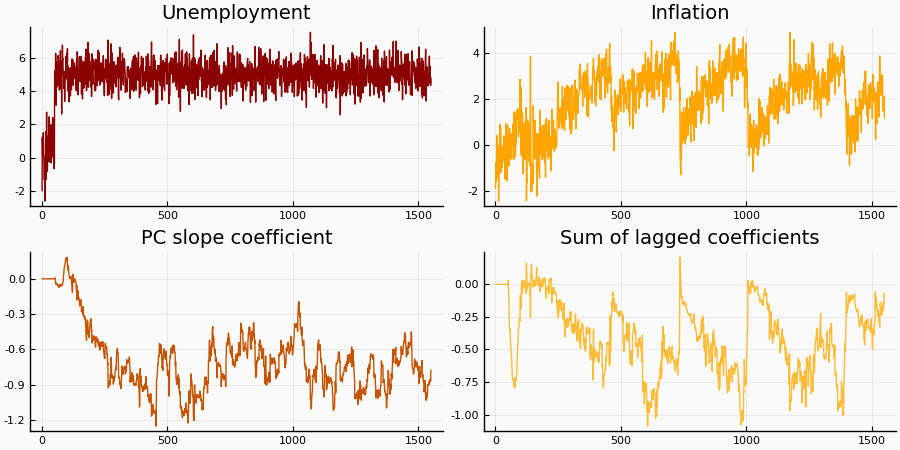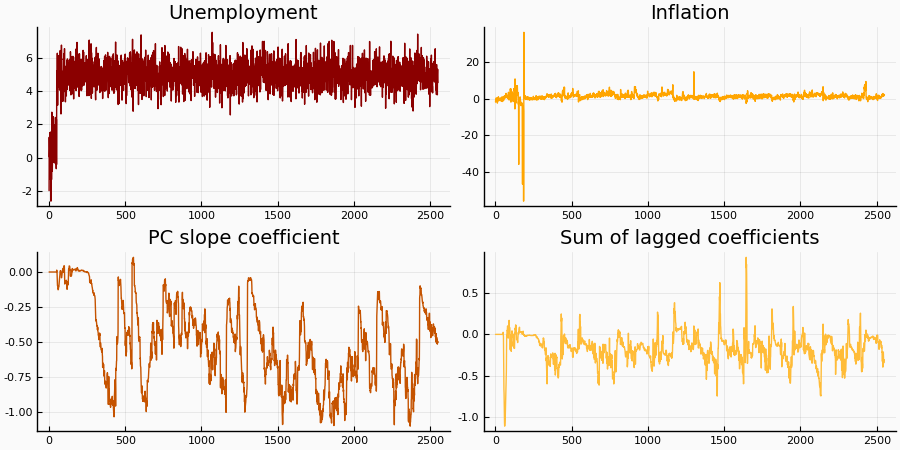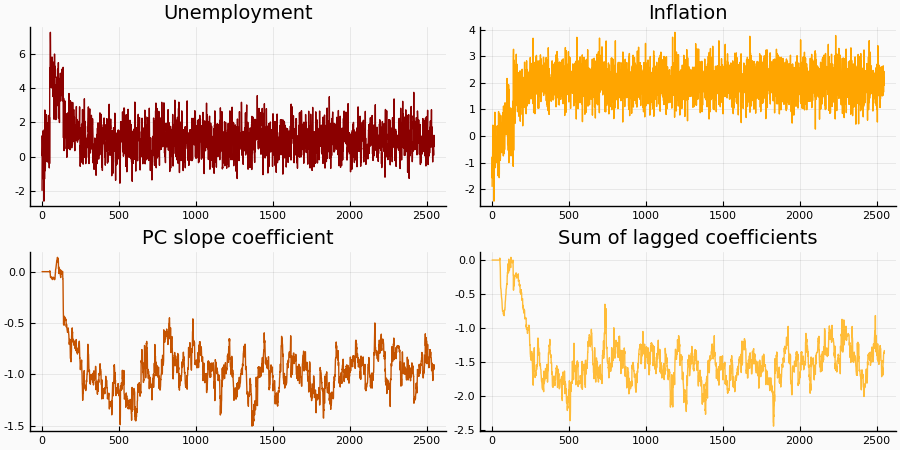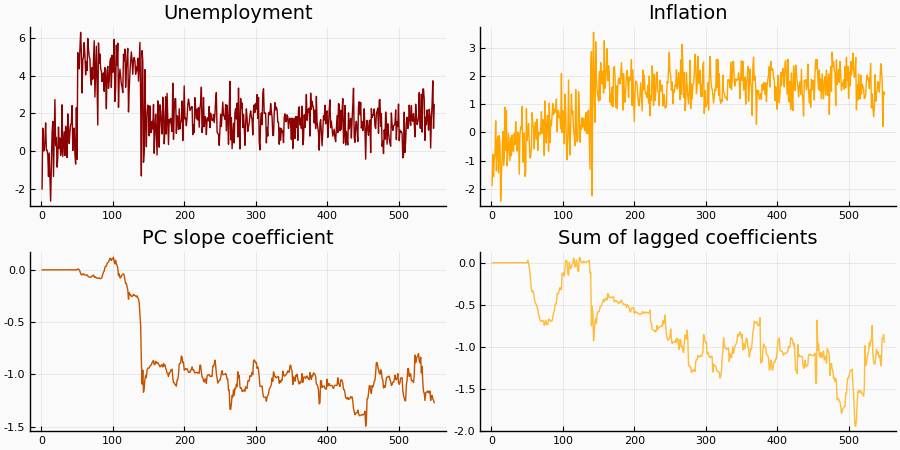# The Conquest of American Inflation¶

## Overview¶

This lecture is loosely based upon Tom Sargent's The Conquest of American Inflation book and makes heavy of the linear-quadratic machinery developed here. Its purpose is to show an example of how an agent whose actions affect the economy might find complications from trying to learn from data generated by policy informed by past inference on the same system. The concepts of self-reference, adaptation, and omitted variables are centrally featured under an innocent surface.

## A simple model¶

The government dislikes inflation $y_t$ and unemployment $U_t$ away from $0$. It seeks to set inflation to minimize

$$L = \sum_{t=0}^\infty \beta^t r_t$$

for

$$r_t = \frac{1}{2}\mathbb{E}\left[ U_t^2 + y_t^2 \right]$$

The only thing it needs is a theory of how inflation and unemployment are linked.

### The true economy¶

In the true economy, unemployment is determined by

$$U_t = U^\star -\theta \left( y_t - x_t \right)$$

where $x_t$ is the mean forecast of inflation by the private sector. $U^\star$ is the "natural" rate of unemployment.

Moreover, we will also make the apparently inocent assumption that inflation $y_t$ is the sum of the government choice of inflation $\hat{y}_t$ plus an iid normally distributed shock $v_t$

### Expectations¶

The plot thickens because the government holds a misspecified model and instead attempts to fit a distributed-lag Phillips curve of the form

$$U_t = \kappa y_t + \gamma' X_t + \epsilon^C_t$$

For most of this lecture we will set $X_t = [U_{t-1}, \dots, U_{t-M_u}, y_{t-1}, \dots, y_{t-M_y}, 1]'$. This means that the government forecasts unemployment with current inflation as well as lagged unemployment, lagged inflation, and a constant term.

Each period, the government updates its estimate of $\kappa$ and $\gamma$. An implementation of recursive least squares is to set

\begin{aligned} \beta_t &= \beta_{t-1} + g_t R^{-1}_{X,t} X_t (U_t - \beta_{t-1}'X_t) \\ R_{X,t} &= R_{X,t-1} + g_t ( X_tX_t' - R_{X,t-1})\\ \end{aligned}

where $\beta_t = [\kappa_t \; \gamma_t']'$. A key parameter is the "gain" parameter $g_t$, which governs the weight to be placed on newer observations. By setting $g_t = \frac{1}{t}$ one recovers the OLS scheme which weighs all observations the same. Setting the gain to a constant (or in general to a non square-summable sequence) causes the estimation to put more weight on recent observations, which is useful if the analyst believes the data-generating process not to be stationary.

The simulation we will run exhibits coefficients that wander as the government changes its mind about how unemployment is determined.

### The private sector's expectations¶

For the private sector, we consider three options.

1. Rational expectations


The simplest case is to make the private sector fully aware of the government's updating scheme and decision process. This amounts to setting $x_t = \hat{y}_t$ at all times.

2. Simple adaptive expectations


This is the 1950's model. Here we make the private sector's new forecast a function of old forecasting errors. That is, $$x_t = x_{t-1} + (1-\lambda) (y_{t-1} - x_{t-1})$$

3. Recursive least squares


Here we endow the private sector with a similar forecasting scheme to the government's. We initialize $g^p_0$ and $R_{X,0}^p$ at (maybe) different points than the government's and may use a different lag structure.

## Problems¶

Can the government ever discover the "true" slope parameter $\theta$? What does the government's model say about the relationship between inflation and unemployment in the short run and the long run? What are implications for the government own choice of inflation?

What are implications of the government's choice of inflation for the evolution of the system from which the government attempts to learn how unemployment is affected by inflation?

Is there a Phillips curve in the data?

## Code¶

To manage the different expectation-formation schemes, the ConquestEconomy type records three different instances of a PLM type. Each PLM instance holds information about the expectations of a particular agent, including those of Nature, also known as the actual law of motion of the system.

In :
using QuantEcon, LinearAlgebra, Random

using Plots
pyplot()

mutable struct PLM
κ::Float64          # Weight on current state (unemployment or inflation)
γ::Vector{Float64}  # Weights on lagged states (unemployment + inflation + constant)

Mu::Int64           # Lags on unemployment
My::Int64           # Lags on inflation

classic::Bool       # TRUE for predicting unemployment with the state, FALSE for predicting inflation

g::Float64          # Gain parameter (only for constant-gain RLS)
R::Matrix{Float64}  # Variance matrix (only for RLS)
end

mutable struct ConquestEconomy
δ::Float64          # Discount factor

plm_g::PLM          # Government's perceived law of motion
plm_p::PLM          # Private sector's perceived law of motion
alm::PLM            # Actual (Nature's perceived) law of motion

private_expectations::String

σe::Float64         # Variance of cost-push shock
σc::Float64         # Variance of inflation control shock
end

lags(plm::PLM) = length(plm.γ)

function sum_coeff(plm::PLM) # Finds the sum of coefficients on (current and) lagged inflation
if plm.classic
current_term = plm.κ
else
current_term = 0
end
return sum(plm.γ[plm.Mu+1:plm.Mu+plm.My]) + current_term
end

get_Ustar(alm::PLM) = alm.γ[end]
get_θ(alm::PLM) = -alm.κ
get_Ustar(ce::ConquestEconomy) = get_Ustar(ce.alm)
get_θ(ce::ConquestEconomy) = get_θ(ce.alm)

function make_ALM(plmg::PLM, plmp::PLM, Ustar::Float64, θ::Float64)
Mu = max(plmg.Mu, plmp.Mu)
My = max(plmg.My, plmp.My)
γ = [zeros(Mu+My); Ustar]
κ = -θ
alm = PLM(κ, γ, Mu, My, true, 0.0, plmg.R, plmg.λ) # In the ALM, all the forecasting variables are irrelevant
return alm
end

make_ALM(ce::ConquestEconomy, Ustar=get_Ustar(ce), θ=get_θ(ce)) = make_ALM(ce.plm_g, ce.plm_p, Ustar, θ)
function update_ALM!(ce::ConquestEconomy)
ce.alm = make_ALM(ce)
nothing
end

function ConquestEconomy(;
δ = 0.98,
θ = 1.0,
g = 0.05,
λ = 0.5,  # λ is the weight on past observations for simple adaptive scheme
σe = 0.5,
σc = 0.5,
Ustar = 5.0,
classic = true,
private_expectations = "rational",

Mu = 1,
My = 1,
Mu_p = 2,
My_p = 2
)

# Set arbitrary coefficients on past inflation and past unemployment
γU = zeros(Mu)
γy = zeros(My)
γ = [γU; γy; 1]
# For the induction hypothesis (weights on y's sum up to zero)
κ = -sum(γy)
R = zeros(Mu+My+1, Mu+My+1)

plm_g = PLM(κ, γ, Mu, My, classic, g, R, λ)

if private_expectations == "RLS"
priv_gain = 0.01
else
Mu_p, My_p, priv_gain = Mu, My, g
end
Rp = zeros(Mu_p+My_p+1, Mu_p+My_p+1)
classic = true # Always use the classical scheme for the private sector
plm_p = PLM(1.0, [zeros(Mu_p+My_p);1], Mu_p, My_p, classic, priv_gain, Rp, λ)

# True process
alm = make_ALM(plm_g, plm_p, Ustar, θ)

return ConquestEconomy(δ, plm_g, plm_p, alm, private_expectations, σe, σc)
end

Out:
ConquestEconomy

#### Some convenience functions to modify expectations schemes on the fly¶

In :
function set_exp!(ce::ConquestEconomy, s::String=""; Mu=1, My=1, Mu_p=2, My_p=2)
if s != "" && s != "rational" && s != "adaptive" && s != "RLS"
println("WARNING: only 'rational', 'adaptive', and 'RLS' expectations allowed.")
else
if s == ""
s = ce.private_expectations
end
ce2 = ConquestEconomy(private_expectations = s, Mu=Mu, My=My, Mu_p=Mu_p, My_p=My_p)
ce.plm_p = ce2.plm_p
ce.plm_g = ce2.plm_g
ce.private_expectations = s
update_ALM!(ce)
end
nothing
end

function set_exp!(ce::ConquestEconomy, n::Int64=0; Mu=1, My=1, Mu_p=2, My_p=2)
if n == 0
set_exp!(ce, "", Mu=Mu, My=My, Mu_p=Mu_p, My_p=My_p)
else
set_exp!(ce, sv[n], Mu=Mu, My=My, Mu_p=Mu_p, My_p=My_p)
end
nothing
end

Out:
set_exp! (generic function with 3 methods)

#### Aside¶

If $M_u$ and $M_y$ are large, the government's problem, which contains $X_t$ in its state, can be difficult to manage. This is why we resort to the immense power of a linear-quadratic formulation.

### Main building block: LQ problems¶

We want to make our problem have the form of choosing controls $u_t$ in state $x_t$ to minimize

$$V(x) = \mathbb{E}[ x'Rx + u'Qu + 2 u'Nx + \beta V(x') ]$$

subject to

$$x' = Ax + Bu + Cw$$

where $w$ are unit-variance iid normal shocks.

#### Setting up our problem¶

The first step is to make $r_t = X_t'RX_t + \hat{y}_t'Q\hat{y}_t + 2 \hat{y}_t'NX_t$. For this, notice that

$$r_t = -\frac{1}{2} \left[ U_t^2 + y_t^2 \right] = -\frac{1}{2} \left[ \left( \kappa y_t + \gamma' X_t + \epsilon^C_t \right)^2 + y_t^2 \right] = -\frac{1}{2} \left[ \kappa^2 y_t^2 + X_t'\gamma\gamma'X_t + 2 X_t'\gamma\kappa y_t + y_t^2 \right] + k$$

where $k$ is a sum of terms that either have zero mean (like those coming from products between endogenous variables and $\epsilon_t^C$) or are independent of policy (like the square term in $\epsilon_t^C$).

This means that we can set $R = \gamma\gamma'$, $Q = [1+\kappa^2]$, and $N = \gamma\kappa$.

Turning to the law of motion, we want to write $X_{t+1} = [U_t,\dots, U_{t-M_u+1}, y_t, \dots, y_{t-M_y+1},1]'$ as $X_{t+1} = AX_t + B \hat{y}_t + C w_{t+1}$. There are three parts: first, we will use the matrix $A$ to transfer lags of endogenous variables from $X_t$ to $X_{t+1}$. Second, we need to record $y_t$ (or equivalently, $\hat{y}_t$) and, finally, the value of $U_t$ that the government expects.

Since the government expects $\mathbb{E}^g[U_t] = \kappa y_t + \gamma'X_t$, we can set the first row of $A$ to coincide with $\gamma'$. We also set the first row of the (one-by-one) matrix $B$ to $\kappa$.

The matrix $A$ is then formed by

\begin{aligned} A = \left( \begin{array}{cccc} [ && \gamma & ] \\ A_1 & 0 & 0 & 0 \\ 0 & 0 & 0 & 0 \\ 0 & 0 & A_2 & 0 \\ 0 & 0 & 0 & 1 \end{array}\right) \end{aligned}

where $A_1$ is $M_u-1 \times M_u-1$ and $A_2$ is $M_y-1\times M_y-1$ and just shift the vectors by sending $U_{t-j}$ from the $j$th place to the $j+1$th place (and accordingly with $y$'s). The $1$ at the end takes care of the constant term (in the last element of $X$). The row of $0$'s (corresponding to $y_t$ in $X_{t+1}$) appears because we will fill the $y_t$ entry using the $B$ matrix.

Because $y_t = \hat{y}_t + v_t$, we can set $B = [\kappa,\dots, 0, 1, 0, \dots, 0]'$ with the $1$ in the $M_u+1$ place. Similarly for matrix $C$, only taking care with the variances

\begin{aligned} C = \left( \begin{array}{cc} \sqrt{\sigma_e} & \kappa \sqrt{\sigma_c} \\ 0 & 0 \\ \dots & \dots \\ 0 & \sqrt{\sigma_c} \\ 0 & 0 \\ \dots & \dots \\ 0& 0 \end{array}\right) \end{aligned}

In :
function make_LQ(plm::PLM, δ, σc, σe)
# Law of motion x_{t+1} = A x_t + B u_t + C w_{t+1}
# u = mean inflation, y = u + σc ϵ

if plm.classic
κ, γ = plm.κ, plm.γ
else
# Invert the Phillips curve in the Keynesian formulation
κ, γ = 1/plm.κ, -plm.γ / plm.κ
end

k = lags(plm)

# Law of motion
A = zeros(k, k)
A[1,:] = γ[:]
for jj in 1:plm.Mu-1
A[jj+1, jj] = 1
end
for jj in 1:plm.My-1
A[plm.Mu+jj+1, plm.Mu+jj] = 1
end
A[end,end] = 1

B = zeros(k,1)
B[1,1] = κ
B[plm.Mu+1,1] = 1

# Shocks: unemployment is affected directly by the cost-push shock and indirectly by the inflation control shock (κ-to-1), inflation affected directly by the control shock
C = zeros(k,2)
C[1,:] = [sqrt(σe) κ*sqrt(σc)]
C[plm.Mu+1, 2] = sqrt(σc)

# Set up objective function
# r = x'Rx + u'Qu + 2 u'Nx

Q = [1+κ^2 for ji in 1:1, jj in 1:1]
R = γ * γ'
N = [κ * γ[jj] for jy in 1:1, jj in 1:k]

lq = QuantEcon.LQ(Q, R, A, B, C, N, bet = δ)
return lq
end

function solve_Phelps!(lq::QuantEcon.LQ)
try
# Use QuantEcon's LQ solver
P, F, d = stationary_values!(lq)

# Bind states
lq.P, lq.F, lq.d = P, F, d

catch
println("Default solver exited. Increasing maxiter.")
# Increase number of iterations by hand
Q, R, A, B, N, C = lq.Q, lq.R, lq.A, lq.B, lq.N, lq.C

# solve Riccati equation, obtain P
A0, B0 = sqrt(lq.bet) * A, sqrt(lq.bet) * B
P = solve_discrete_riccati(A0, B0, R, Q, N, max_it=100)

# Compute F
s1 = Q .+ lq.bet * (B' * P * B)
s2 = lq.bet * (B' * P * A) .+ N
F = s1 \ s2

# Compute d
d = lq.bet * tr(P * C * C') / (1 - lq.bet)

# Bind states
lq.P, lq.F, lq.d = P, F, d
end

nothing
end

Out:
solve_Phelps! (generic function with 1 method)

#### Handling different state spaces for government and private agents¶

A challenge is to keep track of who knows which state. This is where the functions expand_index and expand_matrix come in handy

In :
function expand_index(plm::PLM, alm::PLM, jj::Int64)
new_j = jj
if jj > plm.Mu && jj <= plm.Mu+plm.My
new_j = alm.Mu - plm.Mu + jj
elseif jj == plm.Mu + plm.My + 1
new_j = alm.Mu + alm.My + 1
end
new_j
end

function expand_matrix(plm::PLM, alm::PLM, M::Matrix)
A = zeros(expand_index(plm, alm, size(M,1)), expand_index(plm, alm, size(M,2)))

for ji in 1:size(M,1)
for jj in 1:size(M,2)
A[expand_index(plm, alm, ji), expand_index(plm, alm, jj)] = M[ji,jj]
end
end
A
end

Out:
expand_matrix (generic function with 1 method)

### Updating expectations¶

The functions update_PC_OLS! and update_PC_RLS! run or update the regression in the government's (or the private sector's) expectation scheme. If the government is following the "classical" approach, then the Phillips curve is estimated as unemployment as a function of inflation and lagged variables. Otherwise we estimate the inverted curve as inflation as a function of unemployment and lagged variables.

In :
function simul_PLM(ce::ConquestEconomy, plm::PLM)
lq = make_LQ(plm, ce.δ, ce.σc, ce.σe)

xpath, upath, wpath = compute_sequence(lq, ones(lags(plm)))
end

function update_PC_OLS!(plm::PLM, alm::PLM, Ut, yt, Xt)

if plm.classic
predicted_var = Ut
predictor_var = yt
else
predicted_var = yt
predictor_var = Ut
end

# States has lags on unemployment, lags on inflation, and the constant
States = [Xt[1:plm.Mu]; Xt[alm.Mu+1:alm.Mu+plm.My]; Xt[end]]

# OLS formula U_t = κ y_t + γ'X_t + ϵ_t
X = [predictor_var States']'

# Implement OLS inv(x'x)x'y with x \ y
new_γ = X' \ predicted_var

# Bind coefficients
plm.κ = new_γ
plm.γ = new_γ[2:end]

new_γ
end

function update_PC_RLS!(plm::PLM, alm::PLM, Ut, yt, Xt)

if plm.classic
predicted_var = Ut
predictor_var = yt
else
predicted_var = yt
predictor_var = Ut
end

# States has lags on unemployment, lags on inflation, and the constant
States = [Xt[1:plm.Mu]; Xt[alm.Mu+1:alm.Mu+plm.My]; Xt[end]]

X = [predictor_var States']'

old_γ = [plm.κ; plm.γ[1:end]]

# Use RLS updating formulas
new_R = plm.R + plm.g * (X*X' - plm.R)
new_γ = old_γ + plm.g * (new_R \ (X*(predicted_var - old_γ'*X)))

# Bind new coefficients
plm.κ = new_γ
plm.γ = new_γ[2:end]
plm.R = new_R

new_γ
end

Out:
update_PC_RLS! (generic function with 1 method)

#### Simulation¶

The main function in this code is simul_reest. After initializing the system, it simulates the economy.

Each period, the government chooses inflation $\hat{y}_t$ assuming its current model is the true model of the economy today and in the future. Realized inflation is $\hat{y}_t+\epsilon_t^C$. The private sector simultaneously sets expectations of inflation $x_t$ according to its own forecasting model of the government's choice. Finally, Nature chooses the adjusted natural rate $U^\star + \theta x_t$. Realized unemployment is $U^\star + \theta x_t - \theta y_t + \epsilon_t$.

After these choices have taken place, everyone updates their expectations using the new data they have just seen.

In :
function simul_reest(ce::ConquestEconomy, T=50; OLS::Bool=false)
Random.seed!(1)

# Unpack and prepare
plm_g, plm_p, alm = ce.plm_g, ce.plm_p, ce.alm
expectations = ce.private_expectations
alm_lq = make_LQ(alm, ce.δ, ce.σc, ce.σe)
solve_Phelps!(alm_lq)

# Initial state
xv, uv, wv = simul_PLM(ce, plm_p)
initial_T = 50
xv, uv, wv = xv[:,end-initial_T:end], uv[:,end-initial_T+1:end], wv[:,end-initial_T:end]
xnew = xv[:,end]

# Ensure the constant is a constant (mostly redundant)
xv[end,end] = 1

# Initial variance matrix
yvec = xv[alm.Mu+1, 2:end]
States_vec = vcat(xv[1:plm_g.Mu,1:end-1], xv[alm.Mu+1:alm.Mu+plm_g.My, 1:end-1], xv[end:end, 1:end-1])
X = [yvec States_vec']'
plm_g.γ = zeros(size(plm_g.γ))
plm_g.κ = 0.0
plm_g.R = X*X'

# Initialize private sector
States_vec = vcat(xv[1:plm_p.Mu,1:end-1], xv[alm.Mu+1:alm.Mu+plm_p.My, 1:end-1], xv[end:end, 1:end-1])
X = [yvec States_vec']'
plm_p.R = X*X'
x_priv = 0.0

# Initialize vectors to store results
svec = ones(size(xv,2)-1) * sum_coeff(plm_g)
κvec = ones(size(xv,2)-1) * plm_g.κ
γvec = [plm_g.γ[jj] for jy in 1:1, jj in 1:lags(plm_g)]
πv = vcat(yvec, zeros(T))
Uv = vcat(xv[1, 2:end], zeros(T))

for jj in initial_T+1:initial_T+T
if OLS
plm_g.g = min(plm_g.g, 1/jj)
end

Xt = xnew

# Gov't chooses its action
lq = make_LQ(plm_g, ce.δ, ce.σc, ce.σe)
solve_Phelps!(lq)

# Private sector sets expectations
Fp = zeros(1, lags(plm_p))
if expectations == "adaptive" || expectations == "RLS"
Fp[1,end] = x_priv # Add expected inflation in the constant term
elseif expectations == "rational"
Fp = expand_matrix(plm_g, plm_p, lq.F)
end

# Nature determines the natural rate given current private expectations
θ = get_θ(alm)
Ustar = get_Ustar(alm) + (θ * expand_matrix(plm_p, alm, -Fp) * Xt)
alm_lq.A[1,end] = Ustar

# Gov't implements choice of action

unew = expand_matrix(plm_g, alm, -lq.F) * Xt

# Nature sets the state with the real model
shocks = randn(2)
wnew = alm_lq.C * (shocks .* [sqrt(ce.σe), sqrt(ce.σc)])
xnew = alm_lq.A*xnew + alm_lq.B*unew + wnew

# Save output and unemployment
Ut = xnew
yt = xnew[alm.Mu+1]

# Private sector updates expectations for tomorrow
x_priv = x_priv + (1-plm_p.λ) * ((unew + shocks) - x_priv)
end
if expectations == "RLS"
update_PC_RLS!(plm_p, alm, Ut, yt, xnew)
lq_p = make_LQ(plm_g, ce.δ, ce.σc, ce.σe)
solve_Phelps!(lq_p)

x_priv = (expand_matrix(plm_g, alm, -lq_p.F) * Xt)
end

new_γ = update_PC_RLS!(plm_g, alm, Ut, yt, Xt)
κvec = [κvec; plm_g.κ]
svec = [svec; sum_coeff(plm_g)]
γvec = [γvec; plm_g.γ']

Uv[jj] = Ut
πv[jj] = yt
end

print("Finished simulation with \$expectations expectations")
labels = ["Unemployment" "Inflation" "PC slope coefficient" "Sum of lagged coefficients"]
pl = plot([Uv πv κvec svec], layout=4, title=labels, size = (900,450), color=[2 3 5 8], palette=:heat, bg="#fafafa", ticks=:auto, leg=false)
return pl
end

Out:
simul_reest (generic function with 2 methods)
In :
ce = ConquestEconomy(); set_exp!(ce, 1)
simul_reest(ce, 1500)

Finished simulation with rational expectations
Out:In :
ce = ConquestEconomy(Mu=5,My=7) # With more lags in the government's specification, similar dynamics emerge but the pattern becomes more complicated
simul_reest(ce, 2500)

Finished simulation with rational expectations
Out:In :
ce = ConquestEconomy(); set_exp!(ce, 2)
simul_reest(ce, 2500)

Finished simulation with adaptive expectations
Out:In :
ce = ConquestEconomy(); set_exp!(ce, 3)
simul_reest(ce, 500)

Finished simulation with RLS expectations
Out:In [ ]:


In [ ]: